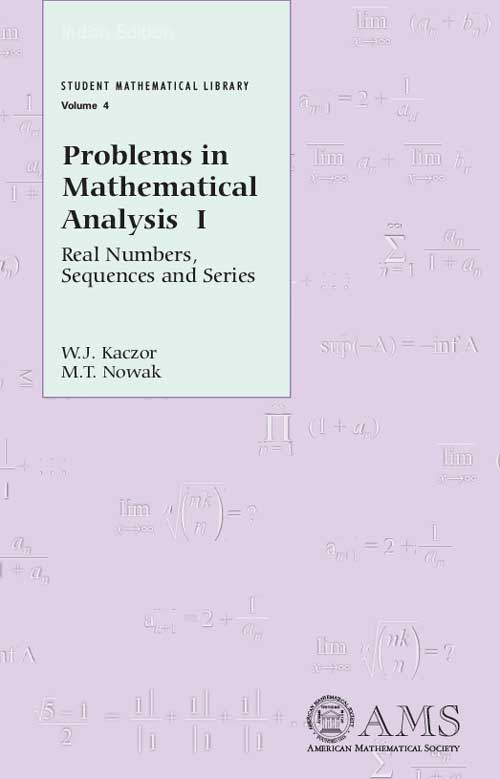Problems in Mathematical Analysis I: Real Numbers, Sequences and Series
W J Kaczor, M T Nowak
Price
1830.00
ISBN
9780821848548
Language
English
Pages
396
Format
Paperback
Dimensions
140 x 216 mm
Year of Publishing
2009
Territorial Rights
Restricted
Imprint
American Mathematical Society
Catalogues

We learn by doing. We learn mathematics by doing problems. And we learn more mathematics by doing more problems. If you want to hone your understanding of continuous and differentiable functions, this book contains hundreds of problems to help you do so. The emphasis here is on real functions of a single variable. Topics include: continuous functions, the intermediate value property, uniform continuity, mean value theorems, Taylors formula, convex functions, sequences and series of functions. The book is mainly geared toward students studying the basic principles of analysis. However, given its selection of problems, organization, and level, it would be an ideal choice for tutorial or problem-solving seminars, particularly those geared toward the Putnam exam. It is also suitable for self-study. The presentation of the material is designed to help student comprehension, to encourage them to ask their own questions, and to start research. The collection of problems will also help teachers who wish to incorporate problems into their lectures. The problems are grouped into sections according to the methods of solution. Solutions for the problems are provided. Problems in Mathematical Analysis I and III are available as Volumes 4 and 21 in the AMS series Student mathematical Library.

W. J. Kaczor and M. T. Nowak, Marie Curie-Sklodowska University, Lublin, Poland

Problems

• Real numbers
• Sequence of real numbers
• Series of real numbers

Solutions

• Real numbers
• Sequences of real numbers
• Series of real numbers
Bibliography
THE BOOKPOINT (INDIA) PVT. LTD.